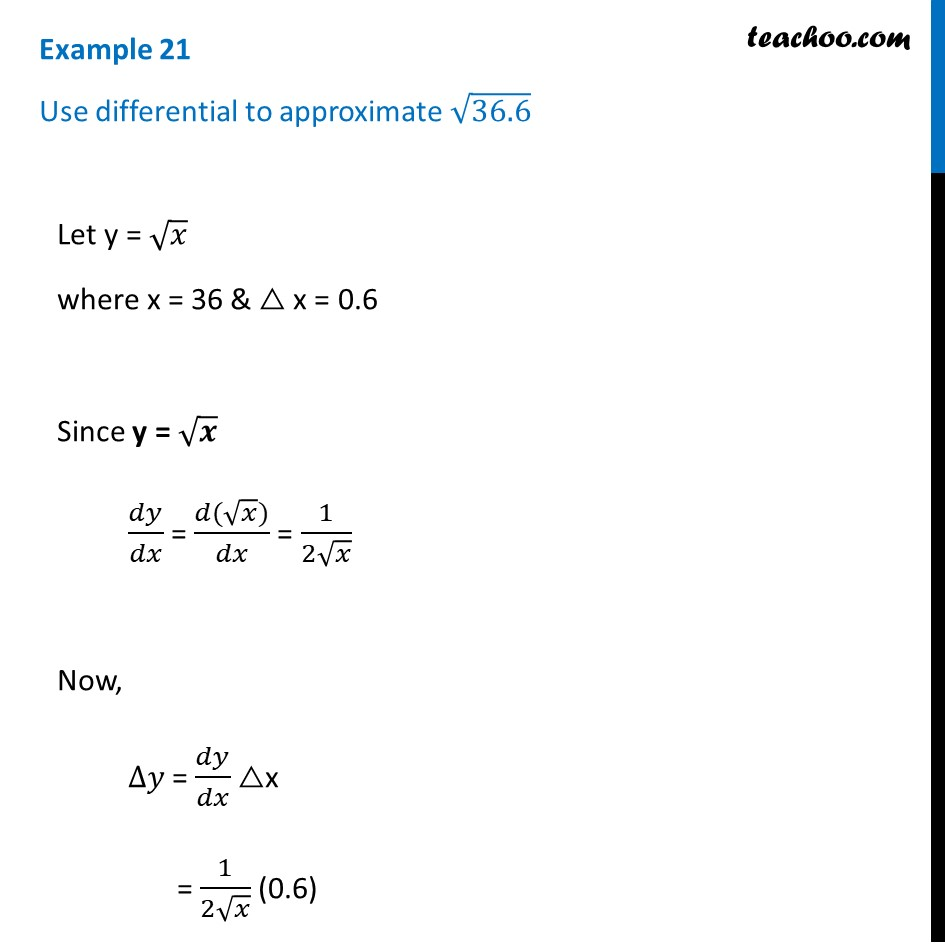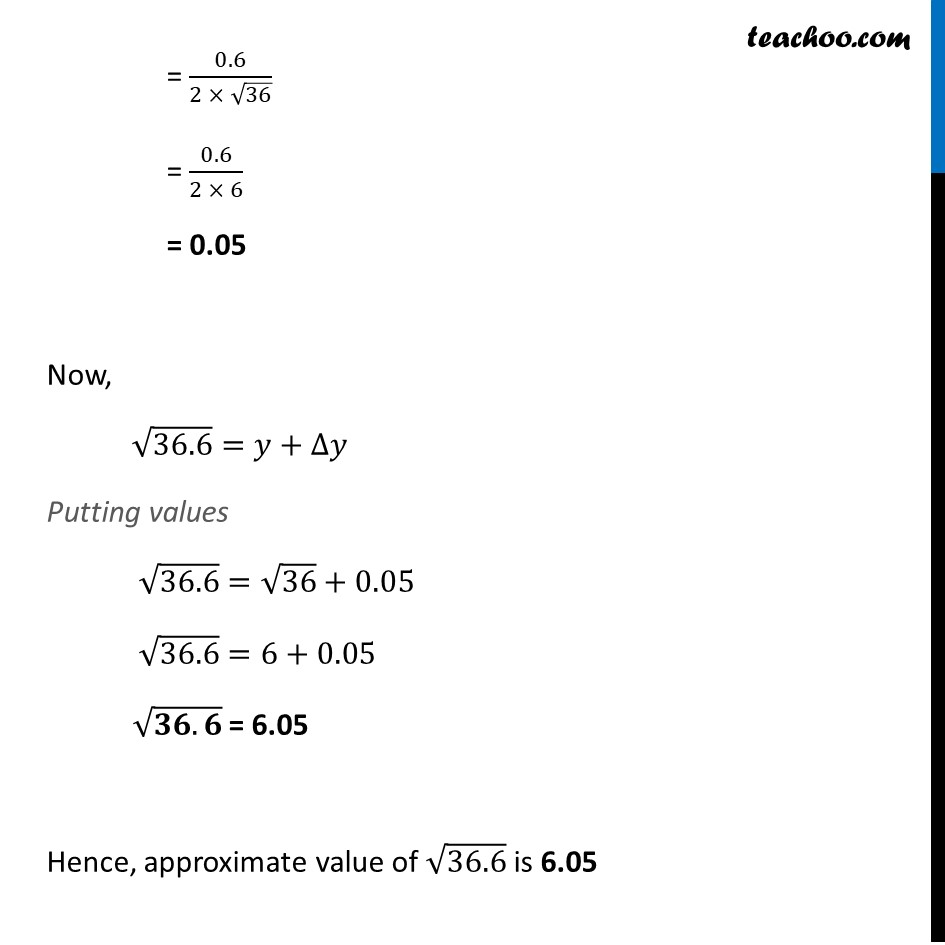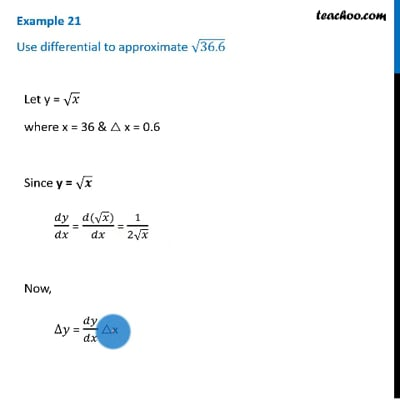Examples

Chapter 6 Class 12 Application of Derivatives
Serial order wiseThis video is only available for Teachoo black users

Solve all your doubts with Teachoo Black (new monthly pack available now!)

### Transcript

Example 21 Use differential to approximate √36.6Let y = √𝑥 where x = 36 & △ x = 0.6 Since y = √𝒙 𝑑𝑦/𝑑𝑥 = (𝑑(√𝑥))/𝑑𝑥 = 1/(2√𝑥) Now, ∆𝑦 = 𝑑𝑦/𝑑𝑥 △x = 1/(2√𝑥) (0.6) = 0.6/(2 × √36) = 0.6/(2 × 6) = 0.05 Now, √36.6=𝑦+∆𝑦 Putting values √36.6=√36+0.05 √36.6=6+0.05 √(𝟑𝟔.𝟔) = 6.05 Hence, approximate value of √36.6 is 6.05